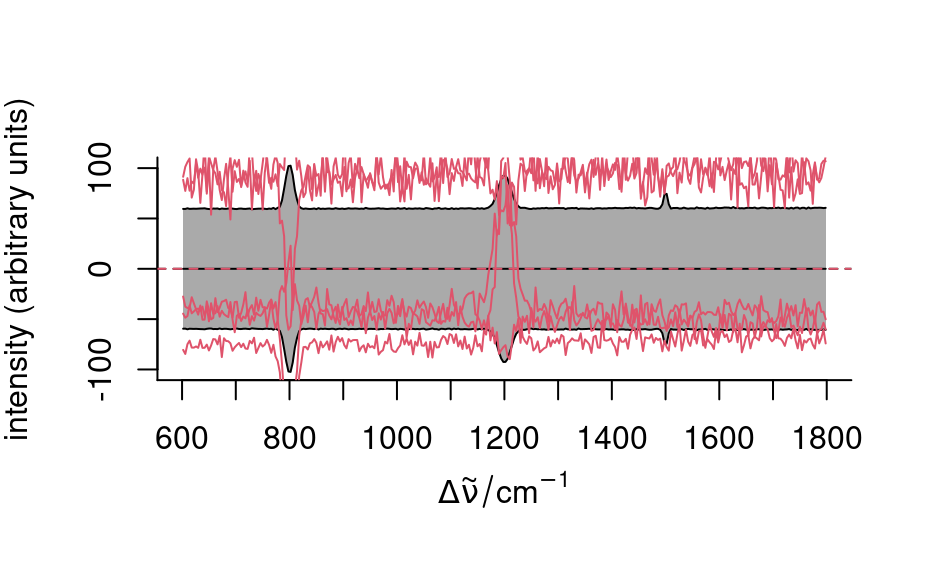Scales the spectra matrix. scale(x, scale = FALSE) centers the data.

# S4 method for hyperSpec
scale(x, center = TRUE, scale = TRUE)

## Arguments

x

the hyperSpec object

center

if TRUE, the data is centered to colMeans(x), FALSE suppresses centering. Alternatively, an object that can be converted to numeric of length nwl(x) by base::as.matrix() (e.g. hyperSpec object containing 1 spectrum) can specify the center spectrum.

scale

if TRUE, the data is scaled to have unit variance at each wavelength, FALSE suppresses scaling. Alternatively, an object that can be converted to numeric of length nwl(x) by base::as.matrix() (e.g. hyperSpec object containing 1 spectrum) can specify the center spectrum.

## Value

the centered & scaled hyperSpec object

## Details

Package scale provides a fast alternative for base::scale()

base::scale()

package scale.

C. Beleites

## Examples


## mean center & variance scale
tmp <- scale(faux_cell)
plot(tmp, "spcmeansd")
plot(sample(tmp, 5), add = TRUE, col = 2)## mean center only
tmp <- scale(faux_cell, scale = FALSE)
plot(tmp, "spcmeansd")
plot(sample(tmp, 5), add = TRUE, col = 2)## custom center
tmp <- sweep(faux_cell, 1, mean, /)
plot(tmp, "spcmeansd")tmp <- scale(tmp, center = quantile(tmp, .05), scale = FALSE)